StatLect

Multinomial coefficient

The multinomial coefficientis used to denote the number of possible partitions of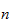objects into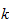groups having numerosity.

The coefficient takes its name from the following multinomial expansion: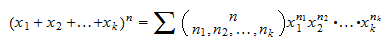where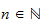and the sum is over all the-tuplessuch that:Example

The number of possible ways to subdivide six objects into three groups of two objects each is given by the multinomial coefficient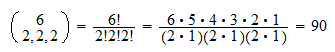More details

The lecture entitled Partitions into groups provides an in-depth presentation of the multinomial coefficient.